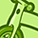# QlikView App Dev

Discussion Board for collaboration related to QlikView App Development.

Announcements
Join us at the Cloud Data and Analytics Tour! REGISTER TODAY
cancel
Showing results for
Search instead for
Did you mean:Creator II

## Quarter average Issue

Hi All,

This could be a simple issue, I tried with multiple expressions but it’s still not working. So I’m here for some help please help me.

I’m just trying to calculate the average in quarter but not getting the correct numbers. Not sure what I’m doing wrong please can someone consider attached excel and qvf to resolve my issue.

Issue:

In the attached qvf for example for Q1-2017 the value is 2.75 – this is wrong

Expected result: (10+11+12)/3 = 11

For Q3-2017- 4.58- wrong

Expected: 17.5

Thank you,

Ramya

1 Solution

Accepted SolutionsMVP

Hi Ramya, the sum expresion needs the field to sum:

Avg(Aggr(Sum({<[Data]={'>0'}>} Data), Year, Month, [Level Data]))

But I'm not sure if this will be ok, in the previous months there are values as zero, if these zero's needs to be counted in the avg you can't ignore zeros.

Another option can be avoid to load from excel recods without any value, to do this you can add this condition to the where clause:

Where YEAR(trim([Date]))&  Num( Month(date(trim([Date]), 'MMMM')), '00')<= text(date(today(),'YYYYMM'))and [Level Data] <> 'All'

and Len(Trim(Data))>0  // if there is no value, it won't be counted for average

;

6 RepliesCreator II
Author

if you get a chance please can some one help with this. Thanks,MVP

Hi Rayma, why you say the expected result for Q3 is 17.5? shouldn't it be 18,3333...?

(20+13+22)/3

You can try with this expression:

Avg(Aggr(Sum([Data]), Year, Month, [Level Data]))

First sums the data of each Month, the does the average of those values.Creator II
Author

Hi Ruben,

Sorry it was my bad, result for Q3 is 17.5? shouldn't it be 18,3333...? yes this is correct. it worked .Thank you,

Regards,

ramyaCreator II
Author

Hi Ruben,

I'm sorry actually for current month there will be no data. So for current quarter (Q3-2017) the calculation should include (july+august)/2.

I have attached the new excel and qvf, i wrote a set expression to exclude '0's but it's not working  please can you help. Thank you so much

RamyaMVP

Hi Ramya, the sum expresion needs the field to sum:

Avg(Aggr(Sum({<[Data]={'>0'}>} Data), Year, Month, [Level Data]))

But I'm not sure if this will be ok, in the previous months there are values as zero, if these zero's needs to be counted in the avg you can't ignore zeros.

Another option can be avoid to load from excel recods without any value, to do this you can add this condition to the where clause:

Where YEAR(trim([Date]))&  Num( Month(date(trim([Date]), 'MMMM')), '00')<= text(date(today(),'YYYYMM'))and [Level Data] <> 'All'

and Len(Trim(Data))>0  // if there is no value, it won't be counted for average

;Creator II
Author

Hi Ruben,

Thank you, i tried the second method it worked.

Ramys